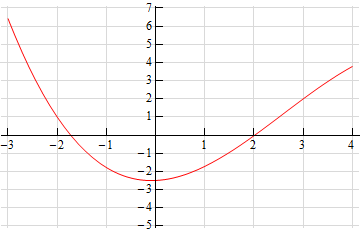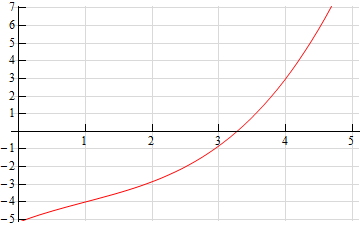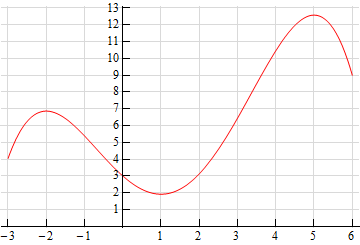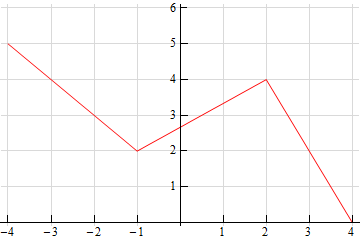Paul's Online Notes
Home / Calculus I / Derivatives / Interpretation of the Derivative
Show Mobile Notice Show All Notes Hide All Notes
Mobile Notice
You appear to be on a device with a "narrow" screen width (i.e. you are probably on a mobile phone). Due to the nature of the mathematics on this site it is best views in landscape mode. If your device is not in landscape mode many of the equations will run off the side of your device (should be able to scroll to see them) and some of the menu items will be cut off due to the narrow screen width.

### Section 3.2 : Interpretation of the Derivative

For problems 1 and 2 use the graph of the function, $$f\left( x \right)$$, estimate the value of $$f'\left( a \right)$$ for the given values of $$a$$.

1. $$a = - 2$$
2. $$a = 3$$Solution
1. $$a = 1$$
2. $$a = 4$$Solution

For problems 3 and 4 sketch the graph of a function that satisfies the given conditions.

1. $$f\left( 1 \right) = 3$$, $$f'\left( 1 \right) = 1$$, $$f\left( 4 \right) = 5$$, $$f'\left( 4 \right) = - 2$$ Solution
2. $$f\left( { - 3} \right) = 5$$, $$f'\left( { - 3} \right) = - 2$$, $$f\left( 1 \right) = 2$$, $$f'\left( 1 \right) = 0$$, $$f\left( 4 \right) = - 2$$, $$f'\left( 4 \right) = - 3$$ Solution

For problems 5 and 6 the graph of a function, $$f\left( x \right)$$, is given. Use this to sketch the graph of the derivative, $$f'\left( x \right)$$.

1.Solution
2.Solution
3. Answer the following questions about the function $$W\left( z \right) = 4{z^2} - 9z$$.
1. Is the function increasing or decreasing at $$z = - 1$$?
2. Is the function increasing or decreasing at $$z = 2$$?
3. Does the function ever stop changing? If yes, at what value(s) of $$z$$ does the function stop changing?
Solution
4. What is the equation of the tangent line to $$f\left( x \right) = 3 - 14x$$ at $$x = 8$$. Solution
5. The position of an object at any time $$t$$ is given by $$\displaystyle s\left( t \right) = \frac{{t + 1}}{{t + 4}}$$.
1. Determine the velocity of the object at any time $$t$$.
2. Does the object ever stop moving? If yes, at what time(s) does the object stop moving?
Solution
6. What is the equation of the tangent line to $$\displaystyle f\left( x \right) = \frac{5}{x}$$ at $$\displaystyle x = \frac{1}{2}$$? Solution
7. Determine where, if anywhere, the function $$g\left( x \right) = {x^3} - 2{x^2} + x - 1$$ stops changing. Solution
8. Determine if the function $$Z\left( t \right) = \sqrt {3t - 4}$$ increasing or decreasing at the given points.
1. $$t = 5$$
2. $$t = 10$$
3. $$t = 300$$
Solution
9. Suppose that the volume of water in a tank for$$0 \le t \le 6$$ is given by $$Q\left( t \right) = 10 + 5t - {t^2}$$.
1. Is the volume of water increasing or decreasing at $$t = 0$$?
2. Is the volume of water increasing or decreasing at $$t = 6$$?
3. Does the volume of water ever stop changing? If yes, at what times(s) does the volume stop changing?
Solution